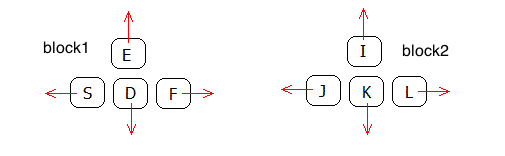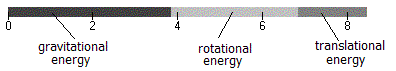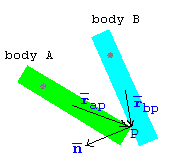# Rigid Body Collisions

previous next
• Sim
• Graph
• Time Graph
• Multi Graph

This simulation uses the Rigid Body Physics Engine to show objects colliding in 2 dimensions.

Click near an object to exert a spring force with your mouse. With the keyboard you can control four "thrusters". The keys S,D,F,E control thrust on block1. The keys J,K,L,I (and also the arrow keys) control thrust on block2. You can also set gravity, elasticity (bounciness), and damping (friction). You can choose from one to six objects. The mass of the green object is adjustable (the others are set to mass 1.0).

Also available are: open source code, documentation and a simple-compiled version which is more customizable.

If you play around with the simulation shown above, you will notice that it can easily get "stuck", which means that objects wind up overlapping. The section below about Resting Contact explains why this happens.

Here is a picture of how the keyboard controls are arranged. If the keys don't work, try clicking near an object first - this ensures that keystrokes are passed to the simulation.keyboard thruster controls

## Energy Bar Graph

Click the "show energy" checkbox to see the bar graph showing the potential, rotational and translational energy.energy bar graph

To check the correctness of the simulation, look at the energy before and after a collision. If damping = 0 and elasticity = 1, then the energy should not change.

See the section on Energy and Momentum for how these quantities are calculated.

## Collision Detection

The explanations here build on those given on the Rigid Body Forces page. In particular, the names of the variables are presented in the section Physics Of Motion for Rigid Bodies

At each step in the simulation, we check to see if there is a collision. The bodies can collide with each other or with a boundary wall. For the rectangular shapes we are using it is simple geometry to determine if a collision has occurred by checking if any vertex is within a wall or foreign body.

When a collision is detected, we use a binary search to back up the simulation to an earlier time just shortly before the collision occurred. We then make the approximation that the collision takes place at this exact time, and calculate the resulting changes in velocity as described below. The Colliding Blocks simulation further describes these aspects of collision handling.

## Physics of Collisions for Rigid Bodies in 2 Dimensions

Handling collisions is the most challenging part of this simulation. The explanation here is fairly condensed, so you may want to read some other descriptions as well.

• Chris Hecker's Rigid Body Dynamics Information. See especially his article for Game Developer Magazine on Collision Response. Easy to read, with many other references listed on the web site. Unfortunately some of the PDF documents do not print their math correctly.
• Physically Based Modeling is a set of course notes from Siggraph '97 by Andrew Witkin and David Baraff. See especially the sections on Rigid Body Dynamics. Fairly complete and rigorous explanations, but still quite readable. Covers the 3 dimensional case and also resting contact forces.
• Physics For Game Developers by David M. Bourg (O'Reilly Press) has a section on linear and angular impulse on page 95, and resting contact forces on page 258. This book has good explanations, but often relies on hard-to-follow programming code to illustrate the physics.
• The textbook Engineering Mechanics Dynamics by Robert Soutas-Little and Daniel Inman has relevant sections on Eccentric Impact.vectors involved in collision

Suppose a vertex on body A is colliding into an edge of body B at the point P. Define the following variables

• ma, mb = mass of bodies A, B
• rap = distance vector from center of mass of body A to point P
• rbp = distance vector from center of mass of body B to point P
• ωa1, ωb1 = initial pre-collision angular velocity of bodies A, B
• ωa2, ωb2 = final post-collision angular velocity of bodies A, B
• va1, vb1 = initial pre-collision velocities of center of mass bodies A, B
• va2, vb2 = final post-collision velocities of center of mass bodies A, B
• vap1 = initial pre-collision velocity of impact point P on body A
• vbp1 = initial pre-collision velocity of impact point P on body B
• vp1 = pre-collision relative velocity of impact points on body A, B
• vp2 = post-collision relative velocity of impact points on body A, B
• n = normal (perpendicular) vector to edge of body B
• e = elasticity (0 = inelastic, 1 = perfectly elastic)

We now use a standard formula for the velocity of an arbitrary point on a rotating and translating rigid body to get the pre-collision velocities of the points of collision (which is the point P on each body).

vap1 = va1 + ωa1 × rap
vbp1 = vb1 + ωb1 × rbp

Similarly we have the final post-collision velocities vap2 and vbp2 as

vap2 = va2 + ωa2 × rap
vbp2 = vb2 + ωb2 × rbp

Here we are regarding the angular velocity as a 3 dimensional vector perpendicular to the plane, so that the cross product is calculated as

ω × r = (0, 0, ω) × (rx, ry, 0) = (−ω ry, ω rx, 0)

Now we can find an expression for the velocity with which the colliding points are approaching each other. We call this the relative velocity at the point P. Let vp1 be the initial (pre-collision) relative velocity and vp2 be the final (post-collision) relative velocity. We define the relative velocities at the point P as follows

vp1 = vap1vbp1
vp2 = vap2vbp2

Using the formulas given above for velocity of a point on a rigid body we can expand these to

 vp1 = va1 + ωa1 × rap − vb1 − ωb1 × rbp (4)
 vp2 = va2 + ωa2 × rap − vb2 − ωb2 × rbp (5)

Let the vector n be normal (perpendicular) to the edge of body B that is being impacted, and pointing outward from body B. Also let the vector n be of length 1. Then we can find the relative velocity in the direction of the normal n by using the vector dot product:

relative normal velocity = vp1 · n

Note that for a collision to occur this relative normal velocity must be negative (that is, the objects must be approaching each other). Let e be the elasticity of the collision, having a value between 0 (inelastic) and 1 (perfectly elastic). We now make an important assumption in the form of the following relation

 vp2 · n = −e vp1 · n (6)

This says that the velocity at which the objects fly apart is proportional to the velocity with which they were coming together. The proportionality factor is the elasticity e .

## Collision Impulse

To resolve the collision, we will use the concept of an impulse. An impulse is the change in momentum of an object when a large force is applied over a very brief period of time. We imagine that during the collision there is a very large force acting for a very brief period of time. If you integrate (sum) that force over that brief time, you get the impulse.

Why do we need this strange concept of an impulse? Why not just use the familiar concept of force as in F = m a ? The answer is that we do not know what the forces are during the collision. With a supercomputer and some very complex software we could model the forces that occur during a collision. We would need to know details about the materials of the bodies, their exact geometry, how they deform under stress, how the stress propagates through the body, etc.

This is far beyond what our simple simulation can do. Luckily, we can assume that the collision happens so quickly that the position and orientation of the bodies do not change during the collision. Instead, all that changes is the velocities of the bodies. Since a change in velocity is a change in momentum (remember momentum = velocity times mass) we have the concept of an impulse.

We are assuming no friction for our collision, so the only force during the collision is in the direction perpendicular to the edge, which is given by the vector n . (Friction would cause a force parallel to the edge as well). Let the net impulse of the collision be j n where j is a parameter to be determined. Body A experiences an impulse of j n while body B experiences the equal but opposite impulse of j n . The impulse is a change in momentum. Momentum has units of velocity times mass, so if we divide the impulse by the mass we get the change in velocity. We can relate pre- and post-collision velocities as

 va2 = va1 + j n / ma (7)
 vb2 = vb1 − j n / mb (8)

The change in angular momentum of body A from the impulse j n is given by rap × j n . You can think of the impulse as being applied at the point P , so it generates an instantaneous torque there. We then divide by the moment of inertia to convert the change in angular momentum to a change in angular velocity, so the post-collision angular velocities are

 ωa2 = ωa1 + (rap × j n) / Ia (9)
 ωb2 = ωb1 − (rbp × j n) / Ib (10)

## Solving for the Impulse Parameter

Now we can put all these various equations together and solve for the impulse parameter j . If you aren't interested in the details of solving the equations just skip down to the expression for j .

We start with equation (6), and then expand using our definition of relative velocity in equations (4-5).

vp2 · n = −e vp1 · n
(vap2vbp2) · n = −e vp1 · n
(va2 + ωa2 × rapvb2ωb2 × rbp) · n = e vp1 · n

Let's expand the left hand side, using the impulse relationships in equations (7-10).

((va1 + j n / ma) + (ωa1 + (rap × j n) / Ia) × rap(vb1j n / mb)(ωb1 − (rbp × j n) / Ib) × rbp) · n = e vp1 · n

We recognize that the left hand side contains the quantity vp1 · n as given by equation (4), so we move that to the right side.

(j n / ma + (rap × j n) × rap / Ia + j n / mb + (rbp × j n) ×rbp / Ib) · n = −(1 + e) vp1 · n

Note that n is assumed to be normalized so that n · n = 1 . Also, to simplify the various vector products, we can use the triple scalar product rule

(A × B) · C = (B × C) · A

to derive the following identity

(A × B) × A · B = (A × B) · (A × B) = (A × B)2

(Here squaring a vector means taking its dot product with itself.) We can then simplify to

j (1 / ma + 1 / mb + (rap × n)2 / Ia + (rbp × n)2 / Ib) = −(1 + e) vp1 · n

Dividing leads to our final expression for j .

 j = −(1 + e) vp1 · n 1⁄ma + 1⁄mb + (rap × n)2 ⁄ Ia + (rbp × n)2 ⁄ Ib
(11)

We can now calculate j at the time of collision using equation (11), and therefore calculate the post-collision velocities using the earlier equations (7-10).

We can use this same expression for calculating collisions with a wall by assuming that the mass of the wall is infinite. So let mb → ∞ and Ib → ∞ and equation (11) becomes

 j = −(1 + e) vap1 · n 1⁄ma + (rap × n)2 ⁄ Ia

## Multiple Impacts

The above analysis only handles the case of a single corner and edge impact. There are several other simultaneous multiple impact cases, such as:

• Two corners of object A impact a single wall
• Two corners of object A impact object B
• Two corners of object A impact different walls
• Two corners of object A impact different objects

If there are simultaneous impacts among unrelated objects, these can be easily handled separately. For example, suppose objects A and B collide at the same time that objects C and D collide, then we can deal with each collision separately because they don't affect each other.

For the case of two adjacent corners of object A impacting either a single wall or another object's edge, we change the impact point to be the midpoint between the two corners and then handle it as other collisions.

The more complicated cases with corners of object A impacting different walls or multiple objects is not handled correctly by this simulation. What the code does is handle each impact separately, which will probably be wrong because these collisions are not independent.

See Collision Handling Methods for a discussion of the pros and cons of various methods.

## Resting Contact

If you play around with the simulation shown above, you will notice that it can easily get "stuck", which means that objects wind up overlapping and the simulation doesn't know how to proceed forward. With elasticity < 1 if you push two objects together with the mouse spring force the simulation will get stuck.

The simulation also gets stuck when gravity > 0 and elasticity < 1 . The problem occurs when an object is settling down onto the floor. The velocity of the object is becoming smaller with each collision because the elasticity is less than one. Eventually the velocity becomes so small that it can't keep gravity from pulling the object down into the floor. This is what results in the simulation getting stuck.

The right thing to do at this point (which the above simulation does not do) is recognize that the object is in resting contact with the floor and add a contact force between the object and the floor. The contact force is calculated to be just enough to prevent the object from penetrating into the floor. See Rigid Body Contact Forces which describes how to do this.

Most all of the "physics engine based" simulations shown on myphysicslab use ContactSim, a class that implements the resting contact calculation in addition to the collision impulse calculations described above.

This web page was first published February 2003.

previous next